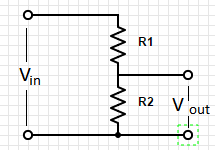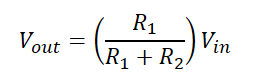# 1.03 Voltage Dividers

Voltage Dividers take an input voltage and gives you a smaller, fractional output voltage. This can be useful for volume control or when interfacing between logic circuits that run at 5V with new/lower power devices that run at 3.3V.

A voltage divider is a simple circuit made up of only two resistors (R1 & R2 in the diagram below).